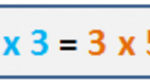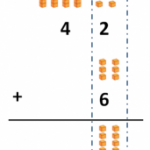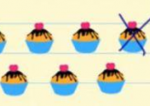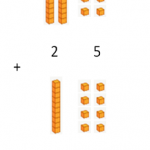Smartick is an advanced online program that teaches kids math and coding in only 15 min. a day

Content about adding and subtracting in elementary mathematics. Exercises, tutorials, and problems with adding and subtracting in elementary mathematics. Here you will find posts about adding and subtracting to make learning mathematics easier and more fun.

Oct04

## Commutative Property in Multiplication and AdditionIn this post, we’re going to see what the commutative property is all about. Example Charles and George learned how to calculate the area of a rectangle in math class by using the base by height formula. Each of them was given a problem using the same rectangle, asking them to calculate the area. Charles […]

Sep13

## Learn and Practice Vertical AddingIn today’s post, we are going to review vertical adding. As always, we are going to review with examples that help us understand what it consists of and how it’s done. But before starting, let’s review the math cubes that we’re going to use in order to add. How do we represent a number with […]

Aug25

## Learn How to Subtract with ExamplesIn this post, we are going to learn how to subtract. First things first, we are going to learn exactly what subtraction is. Subtraction is taking away a certain amount from another amount that we already have. Some examples of how to subtract: If we have 5 purple balls in a box, and we take […]

Aug12

## How to Solve an Addition Problem with RegroupingDo you know how to solve an addition problem with regrouping? Do you know all the steps and why they’re important? Sometimes you learn how to solve math problems, but you don’t understand why you solve them in a particular way. This is why we are going to review how to solve an addition problem […]

Aug04We will learn about the addition properties through an everyday situation: Preparing a backpack for school. Shall we begin? We look for an empty backpack and open it. First, we put in 3 large notebooks and then 6 small notebooks. How many notebooks are there in the bag? To answer this question, simply add the […]

Jul22

## Finding the Average and the Median

In today’s post, we’re going to learn two basic concepts of statistics: average and median. Imagine if we asked all of our classmates what grade their math grade was for this marking period. Assuming that there are 25 people in our class, we’ll collect 25 numbers: It’s a little complicated to process the numbers if […]

Jul08

## Practice Adding Fractions with Examples

Today, we’re going to walk through some examples of adding fractions. Before you read this post, you might want to check out a previous post where we explain how to add fractions step by step. Let’s begin with the simplest of examples: Adding Fractions with the Same Denominator For example: The only thing we need […]

Jul06

## How to Add with and without Regrouping

In this post, we are going to learn how to do addition problems with and without regrouping. How to Add without Regrouping Place the addends one on top of the other so that the place values fall in the same columns. Add each column together separately, starting with the 1s place. The sums go below each column, […]